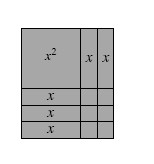### Home > AC > Chapter 4 > Lesson 4.2.2 > Problem4-81

4-81.

Examine the rectangle formed with algebra tiles below.

1. Find the area of the entire rectangle. That is, what is the sum of the areas of the algebra tiles?

Group the pieces that have equal areas, then sum the groups.

$x² + 5x + 6$

1. Find the perimeter of the entire rectangle. Show all work.

Find the lengths of the individual sides.

Sum the sides to find the total perimeter.